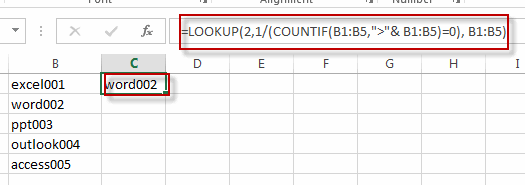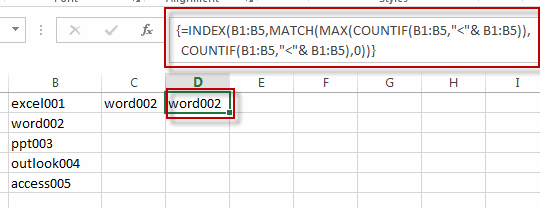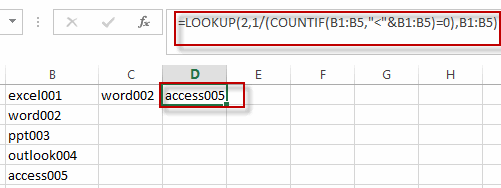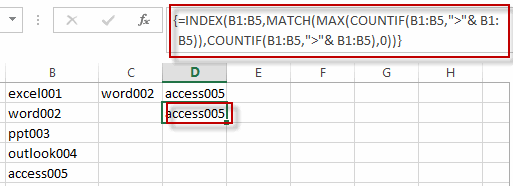# Finding the Max and Min value in an Alphanumeric Data

This post will guide you how to find the maximal and minimal value or string from an alphanumeric data list in excel. How do I retrieve or get the max or min string value based on alphabetic order from a string data in excel.

For example, if you want to find the Maximal or minimal string value from an alphanumeric data list in the range B1:B5, you can create a formula based on the LOOKUP function and the COUNTIF function.

To get the max value based on the alphabetic order, you can use the following formula:

`=LOOKUP(2,1/(COUNTIF(B1:B5,">"& B1:B5)=0), B1:B5)`You can also use the following array formula to achieve the same result. Just follow these steps to apply the array formula:

#1 type the formula in the formula box of cell D1

=INDEX(B1:B5,MATCH(MAX(COUNTIF(B1:B5,”<“& B1:B5)),COUNTIF(B1:B5,”<“& B1:B5),0))

#2 Press Ctrl + Shift +Enter on your keyboard to make the formula as an array formula.

#3 the formula will be change as the following style:

`{=INDEX(B1:B5,MATCH(MAX(COUNTIF(B1:B5,"<"& B1:B5)),COUNTIF(B1:B5,"<"& B1:B5),0))}`

#4 let’s see the result.To get the min value based on the alphabetic order, you can use the following formula:

`=LOOKUP(2,1/(COUNTIF(B1:B5,"<"&B1:B5)=0),B1:B5)`Of course, you can also use another array formula to get the minimal value based on alphabetic order, just refer to the above steps to apply the following array formula.

`{=INDEX(B1:B5,MATCH(MAX(COUNTIF(B1:B5,">"& B1:B5)),COUNTIF(B1:B5,">"& B1:B5),0))}`### Related Functions

• Excel LOOKUP function
The Excel LOOKUP function will search a value in a vector or array.The syntax of the LOOKUP function is as below:= LOOKUP (lookup_value, lookup_vector, [result_vector])…
• Excel COUNTIF function
The Excel COUNTIF function will count the number of cells in a range that meet a given criteria. This function can be used to count the different kinds of cells with number, date, text values, blank, non-blanks, or containing specific characters.etc.= COUNTIF (range, criteria)…
• Excel INDEX function
The Excel INDEX function returns a value from a table based on the index (row number and column number)The INDEX function is a build-in function in Microsoft Excel and it is categorized as a Lookup and Reference Function.The syntax of the INDEX function is as below:= INDEX (array, row_num,[column_num])…
• Excel MATCH  function
The Excel MATCH function search a value in an array and returns the position of that item.The MATCH function is a build-in function in Microsoft Excel and it is categorized as a Lookup and Reference Function.The syntax of the MATCH function is as below:= MATCH  (lookup_value, lookup_array, [match_type])….
• Excel MAX function
The Excel MAX function returns the largest numeric value from the numbers that you provided. Or returns the largest value in the array.= MAX(num1,[num2,…numn])…
Related Posts

How to Find the Earliest and Latest Date in Excel

We have a range of dates and we want to look up the earliest and the latest date based on certain criteria like the earliest date for a showing movie, we can use MIN and MAX functions with IF function ...

How to Create Dynamic Drop Down List without Blank in Excel

This post will guide you how to create dynamic drop down list without blank cells in Microsoft Excel. In Excel, and you can use Data Validation feature to improve the efficiency of data entry in excel, and it also be ...

How to Calculate the Average Excluding the Smallest & Highest Numbers in Excel

Calculating the average for a batch of data is frequently used in our daily life. But for some cases like statistic the average score in a competition, or price analysis, we often calculate the average excluding the smallest and highest ...

How to Get the Maximum or Minimum Absolute Value in Excel

It is easy to find the maximum or minimum value in a batch of data in excel, but if this batch of numbers contains both positive and negative numbers, the maximum or minimum absolute value cannot be found out by ...

How to Find the Largest Value in a Column and Return the Adjacent Cell Value in Excel

This post will guide you how to find the largest value in a column and then return the adjacent cell value with a formula in Excel. How do I find the max value and return the cell value from the ...

How to Extract the Column Header of the Largest Value in a Row in Excel

This post will guide you how to find the largest value in a row and return column header in Excel. How to retrieve the column header of the largest value in a row with a formula in Excel. How to ...

How to Find Max And Min Value with Single or Multiple Criteria in Excel

This post will guide you how to find the maximum value in a range of cells based on single or multiple criteria in Excel. How do I calculate the Minimum value based on criteria with MIN Function in Excel. Find ...

How to Check If value Is between Two Numbers in Excel

This post will guide you how to check if a cell value is between two numbers in Excel. How do I build an IF statement that test if a cell value is between two given values in formula in Excel. ...

How to Get Cell Address of Max or Min Value in a Range in Excel

This post will guide you how to return the cell address of a maximum cell value from a given range of cells with a formula in Excel. How do I Find the minimum value from a given range and return ...

How to Highlight The Highest and Lowest Value in Each Row or Column in Excel

This post will guide you how to highlight the highest value in each row or column in Excel. How do I highlight the lowest value in each row and column with conditional formatting function. Highlight Highest Value in Each Row ...

Sidebar